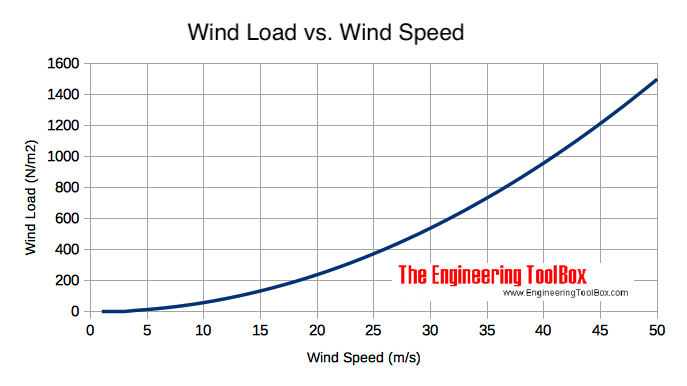Engineering ToolBox - Resources, Tools and Basic Information for Engineering and Design of Technical Applications!

# Dynamic Pressure

## Dynamic pressure is the kinetic energy per unit volume of a fluid in movement.

Dynamic pressure is the kinetic energy of a flowing fluid - liquid or gas - per unit volume - and can be expressed as

pd = 1/2 ρ v2                        (1)

where

pd = dynamic pressure (N/m2 (Pa), lbf/ft2 (psf))

ρ = density of fluid (kg/m3, slugs/ft3)

v = velocity (m/s, ft/s)

### Dynamic Pressure Calculator - SI Units

The default values below are for water with density of 1000 kg/m3.

ρ - density of fluid (kg/m3)

v - velocity (m/s)

• 1 N/m2 = 1 Pa = 1.4504x10-4 lbf/in2 (psi) = 0.02089 lbf/ft2 (psf) = 1x10-5 bar = 4.03x10-3 in water = 0.336x10-3 ft water = 0.1024 mm water = 0.295x10-3 in mercury = 7.55x10-3 mm mercury = 0.1024 kp/m2 = 0.993x10-5 atm

### Dynamic Pressure Calculator - Imperial Units

The default values below are for water with density of 1.940 slugs.

ρ - density of fluid (slugs/ft3)

v - velocity (ft/s)

Some common densities at atmospheric pressure:

• Water - 0oC - 1000 kg/m3
• Water - 32oF - 1.940 slugs/ft3
• Air - 20oC - 1.2 kg/m3
• Air - 60oF - 2.373 10-3 slugs/ft3

### Example - The Dynamic Pressure in a Water Flow

The dynamic pressure in water with - temperature 20oC - density 1000 kg/m3 and velocity 5 m/s - can be calculated as

pd = 1/2 (1000 kg/m3) (5 m/s)2

= 12500 Pa

= 12.5 kPa

### Example - Hurricane and Force acting on a Wall

The dynamic pressure in a hurricane with air temperature 20oC, density of air 1.2 kg/m3 and wind speed 37 m/s can be calculated as

pd = 1/2 (1.2 kg/m3) (37 m/s)2

= 821 Pa (N/m2)

The force acting directly on a wall with area 10 m2 can be calculated as

F = pd A

= (821 N/m2) (10 m2)

= 8210 N

= 8.2 kN

- almost the weight of a small car.

Note! - the real force acting on the wall - or an other obstruction in the wind - is in general more complicated to calculate due to drag, turbulence and other effects.## Related Topics

• ### Fluid Mechanics

The study of fluids - liquids and gases. Involving velocity, pressure, density and temperature as functions of space and time.

## Related Documents

• ### Air Curtains or Air Screens

Calculate air curtains or air screens in open doorways used to keep acceptable indoor comfort in buildings.
• ### Beaufort Wind Scale

The Beaufort description and observation scale for wind and wind speed.
• ### Distance Traveled vs. Speed and Time - Calculator and Chart

Velocity plotted in time used diagram.
• ### Ducts Sizing - the Velocity Reduction Method

The velocity reduction method can be used when sizing air ducts.
• ### Kinetic Energy

Energy possessed by an object's motion is kinetic energy.
• ### Pitot Tubes

Pitot tubes can be used to measure fluid flow velocities by measuring the difference between static and dynamic pressure in the flow.

## Engineering ToolBox - SketchUp Extension - Online 3D modeling!

Add standard and customized parametric components - like flange beams, lumbers, piping, stairs and more - to your Sketchup model with the Engineering ToolBox - SketchUp Extension - enabled for use with older versions of the amazing SketchUp Make and the newer "up to date" SketchUp Pro . Add the Engineering ToolBox extension to your SketchUp Make/Pro from the Extension Warehouse !

We don't collect information from our users. More about

## Citation

• The Engineering ToolBox (2008). Dynamic Pressure. [online] Available at: https://www.engineeringtoolbox.com/dynamic-pressure-d_1037.html [Accessed Day Month Year].

Modify the access date according your visit.

9.19.12